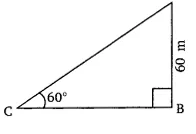# 5. A kite is flying at a height of 60 m above the ground. The string attached to the kite is temporarily tied to a point on the ground. The inclination of the string with the ground is 60°. Find the length of the string, assuming that there is no slack in the string.

M manish

AGiven that,
The length of AB = 60 m and the inclination of the string with the ground at point C is .
Let the length of the string AC be .
According to question,
In right triangle CBA,

The value of length of the string ()  is  = 40(1.732) = 69.28 m
Hence the length of the string is 69.28 m.

Exams
Articles
Questions